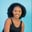Related Tags

python3
numpy

# How to compute the absolute values of a 2D array in PythonMaria Elijah

### Overview

In Python, the absolute() method from the numpy module is used to find the absolute values of a NumPy array. This method computes the absolute values of a complex array input. This is done element-wise.

Note: In Python, a list of lists can be used to generate a two-dimensional (2D) array.

### Syntax

numpy.absolute(arr,out=None, where=True,casting='same_kind', order='K', dtype=None,subok=True[, signature, extobj])
-
<ufunc absolute'>)


### Parameters

• arr: This represents the input array.

• out: This specifies where the result is stored. This is an optional parameter.

• where: This represents the condition in which the input gets broadcasted. This is an optional parameter.

• casting: This specifies the kind of casting that is allowed. The value may be no, equiv, safe, same_kind, or unsafe. This is an optional parameter.

• order: This defines the output array’s memory layout and calculation iteration order. Its default value is K.

• C indicates that the output should be C-contiguous.
• F indicates that it should be F-contiguous.
• A indicates that it should be F-contiguous if the inputs are F-contiguous but not also C-contiguous. Otherwise, C-contiguous.
• K indicates that it should closely match the element ordering of the inputs.
• subok: If False is specified, it returns a strict array rather than a subtype. The default is True.

• dtype: It modifies the DType of the output arrays. Its default value is None.

### Return value

This method returns absolute values of an array.

Note: This function is abbreviated as np.abs.

### Example

# import numpy
import numpy as np

# create 2D array using np.array
arr = np.array([[-7,-3.4,1.2 + 1j], [-2,3 + 1j,-9.5]])

print('Abs Arr:', np.absolute(arr), sep='\n')
print('Org Arr:', arr, sep='\n')

print('Abs Arr:', np.absolute(arr, out=arr), sep='\n')
print('Org Arr:', arr, sep='\n')

### Explanation

• Line 5: We create a 2D array called arr using the list of lists, which is then passed as an argument to the method np.array() to convert it to a numpy array.
• Line 7: We use the np.absolute() method to calculate the absolute values of the input array, arr.
• Line 10: We not only use the np.absolute() method to calculate the absolute values of the input array arr, but also store the results in the same array.

RELATED TAGS

python3
numpy

CONTRIBUTORMaria Elijah
RELATED COURSES

View all Courses

Keep Exploring

Learn in-demand tech skills in half the time